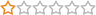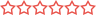## CONDITIONAL FIELDS

• DAVIDPL
• Topic Author
• Offline
• Fresh Boarder
•• Posts: 16

### DAVIDPL replied the topic: CONDITIONAL FIELDS

I have not been able to make it work with the code you have indicated to me maybe I am doing something wrong
• mihaela
• Offline
•• Posts: 2969
• Karma: 100

### mihaela replied the topic: CONDITIONAL FIELDS

Hello again,

Maybe it would the best if you sent me backend login info so I can look into why is this not working from the first hand.

Which solution have you used, the one with Actionscript or Initialization script?

Regards,

Mihaela
• DAVIDPL
• Topic Author
• Offline
• Fresh Boarder
•• Posts: 16

### DAVIDPL replied the topic: CONDITIONAL FIELDS

Hi Mihaela
i have used accionscript
Fortunately everything has gone perfectly, I have resorted to using code for each of the actions, then I indicate the final code that works well.

código final:
function ff_Homologacion_de_club_init()
{ setInterval(calc, 500);
} //ff_Homologacion_de_club_init

function calc() {
var vdeporte1d = (ff_getElementByName('1_deporte').value);
var vdeporte2d = (ff_getElementByName('2_deporte').value);
var vdeporte3d = (ff_getElementByName('3_deporte').value);
var vdeporte4d = (ff_getElementByName('4_deporte').value);
var vdeporte5d = (ff_getElementByName('5_deporte').value);

if (vdeporte1d == "0") {
ff_getElementByName('Importe1D').value= "0"; ff_getElementByName('Total').value = (Number(ff_getElementByName('Importe1D').value) + Number(ff_getElementByName('Importe2D').value) + Number(ff_getElementByName('Importe3D').value) + Number(ff_getElementByName('Importe4D').value) + Number(ff_getElementByName('Importe5D').value));
}
if (vdeporte1d == "1") {
ff_getElementByName('Importe1D').value= "231"; ff_getElementByName('Total').value = (Number(ff_getElementByName('Importe1D').value) + Number(ff_getElementByName('Importe2D').value) + Number(ff_getElementByName('Importe3D').value) + Number(ff_getElementByName('Importe4D').value) + Number(ff_getElementByName('Importe5D').value));
}
if (vdeporte1d == "2") {
ff_getElementByName('Importe1D').value= "231"; ff_getElementByName('Total').value = (Number(ff_getElementByName('Importe1D').value) + Number(ff_getElementByName('Importe2D').value) + Number(ff_getElementByName('Importe3D').value) + Number(ff_getElementByName('Importe4D').value) + Number(ff_getElementByName('Importe5D').value));
}
if (vdeporte1d == "3") {
ff_getElementByName('Importe1D').value= "231"; ff_getElementByName('Total').value = (Number(ff_getElementByName('Importe1D').value) + Number(ff_getElementByName('Importe2D').value) + Number(ff_getElementByName('Importe3D').value) + Number(ff_getElementByName('Importe4D').value) + Number(ff_getElementByName('Importe5D').value));
}
if (vdeporte1d == "4") {
ff_getElementByName('Importe1D').value= "231"; ff_getElementByName('Total').value = (Number(ff_getElementByName('Importe1D').value) + Number(ff_getElementByName('Importe2D').value) + Number(ff_getElementByName('Importe3D').value) + Number(ff_getElementByName('Importe4D').value) + Number(ff_getElementByName('Importe5D').value));
}
if (vdeporte1d == "5") {
ff_getElementByName('Importe1D').value= "231"; ff_getElementByName('Total').value = (Number(ff_getElementByName('Importe1D').value) + Number(ff_getElementByName('Importe2D').value) + Number(ff_getElementByName('Importe3D').value) + Number(ff_getElementByName('Importe4D').value) + Number(ff_getElementByName('Importe5D').value));
}
if (vdeporte1d == "6") {
ff_getElementByName('Importe1D').value= "231"; ff_getElementByName('Total').value = (Number(ff_getElementByName('Importe1D').value) + Number(ff_getElementByName('Importe2D').value) + Number(ff_getElementByName('Importe3D').value) + Number(ff_getElementByName('Importe4D').value) + Number(ff_getElementByName('Importe5D').value));
}
if (vdeporte2d == "0") {
ff_getElementByName('Importe2D').value= "0"; ff_getElementByName('Total').value = (Number(ff_getElementByName('Importe1D').value) + Number(ff_getElementByName('Importe2D').value) + Number(ff_getElementByName('Importe3D').value) + Number(ff_getElementByName('Importe4D').value) + Number(ff_getElementByName('Importe5D').value));
}
else if (vdeporte2d == "1") {
ff_getElementByName('Importe2D').value= "52"; ff_getElementByName('Total').value = (Number(ff_getElementByName('Importe1D').value) + Number(ff_getElementByName('Importe2D').value) + Number(ff_getElementByName('Importe3D').value) + Number(ff_getElementByName('Importe4D').value) + Number(ff_getElementByName('Importe5D').value));
}
else if (vdeporte2d == "2") {
ff_getElementByName('Importe2D').value= "52"; ff_getElementByName('Total').value = (Number(ff_getElementByName('Importe1D').value) + Number(ff_getElementByName('Importe2D').value) + Number(ff_getElementByName('Importe3D').value) + Number(ff_getElementByName('Importe4D').value) + Number(ff_getElementByName('Importe5D').value));
}
else if (vdeporte2d == "3") {
ff_getElementByName('Importe2D').value= "52"; ff_getElementByName('Total').value = (Number(ff_getElementByName('Importe1D').value) + Number(ff_getElementByName('Importe2D').value) + Number(ff_getElementByName('Importe3D').value) + Number(ff_getElementByName('Importe4D').value) + Number(ff_getElementByName('Importe5D').value));
}
else if (vdeporte2d == "4") {
ff_getElementByName('Importe2D').value= "52"; ff_getElementByName('Total').value = (Number(ff_getElementByName('Importe1D').value) + Number(ff_getElementByName('Importe2D').value) + Number(ff_getElementByName('Importe3D').value) + Number(ff_getElementByName('Importe4D').value) + Number(ff_getElementByName('Importe5D').value));
}
else if (vdeporte2d == "5") {
ff_getElementByName('Importe2D').value= "52"; ff_getElementByName('Total').value = (Number(ff_getElementByName('Importe1D').value) + Number(ff_getElementByName('Importe2D').value) + Number(ff_getElementByName('Importe3D').value) + Number(ff_getElementByName('Importe4D').value) + Number(ff_getElementByName('Importe5D').value));
}
else if (vdeporte2d == "6") {
ff_getElementByName('Importe2D').value= "52"; ff_getElementByName('Total').value = (Number(ff_getElementByName('Importe1D').value) + Number(ff_getElementByName('Importe2D').value) + Number(ff_getElementByName('Importe3D').value) + Number(ff_getElementByName('Importe4D').value) + Number(ff_getElementByName('Importe5D').value));
}
if (vdeporte3d == "0") {
ff_getElementByName('Importe3D').value= "0"; ff_getElementByName('Total').value = (Number(ff_getElementByName('Importe1D').value) + Number(ff_getElementByName('Importe2D').value) + Number(ff_getElementByName('Importe3D').value) + Number(ff_getElementByName('Importe4D').value) + Number(ff_getElementByName('Importe5D').value));
}
else if (vdeporte3d == "1") {
ff_getElementByName('Importe3D').value= "52"; ff_getElementByName('Total').value = (Number(ff_getElementByName('Importe1D').value) + Number(ff_getElementByName('Importe2D').value) + Number(ff_getElementByName('Importe3D').value) + Number(ff_getElementByName('Importe4D').value) + Number(ff_getElementByName('Importe5D').value));
}
else if (vdeporte3d == "2") {
ff_getElementByName('Importe3D').value= "52"; ff_getElementByName('Total').value = (Number(ff_getElementByName('Importe1D').value) + Number(ff_getElementByName('Importe2D').value) + Number(ff_getElementByName('Importe3D').value) + Number(ff_getElementByName('Importe4D').value) + Number(ff_getElementByName('Importe5D').value));
}
else if (vdeporte3d == "3") {
ff_getElementByName('Importe3D').value= "52"; ff_getElementByName('Total').value = (Number(ff_getElementByName('Importe1D').value) + Number(ff_getElementByName('Importe2D').value) + Number(ff_getElementByName('Importe3D').value) + Number(ff_getElementByName('Importe4D').value) + Number(ff_getElementByName('Importe5D').value));
}
else if (vdeporte3d == "4") {
ff_getElementByName('Importe3D').value= "52"; ff_getElementByName('Total').value = (Number(ff_getElementByName('Importe1D').value) + Number(ff_getElementByName('Importe2D').value) + Number(ff_getElementByName('Importe3D').value) + Number(ff_getElementByName('Importe4D').value) + Number(ff_getElementByName('Importe5D').value));
}
else if (vdeporte3d == "5") {
ff_getElementByName('Importe3D').value= "52"; ff_getElementByName('Total').value = (Number(ff_getElementByName('Importe1D').value) + Number(ff_getElementByName('Importe2D').value) + Number(ff_getElementByName('Importe3D').value) + Number(ff_getElementByName('Importe4D').value) + Number(ff_getElementByName('Importe5D').value));
}
else if (vdeporte3d == "6") {
ff_getElementByName('Importe3D').value= "52"; ff_getElementByName('Total').value = (Number(ff_getElementByName('Importe1D').value) + Number(ff_getElementByName('Importe2D').value) + Number(ff_getElementByName('Importe3D').value) + Number(ff_getElementByName('Importe4D').value) + Number(ff_getElementByName('Importe5D').value));
}
if (vdeporte4d == "0") {
ff_getElementByName('Importe4D').value= "0"; ff_getElementByName('Total').value = (Number(ff_getElementByName('Importe1D').value) + Number(ff_getElementByName('Importe2D').value) + Number(ff_getElementByName('Importe3D').value) + Number(ff_getElementByName('Importe4D').value) + Number(ff_getElementByName('Importe5D').value));
}
else if (vdeporte4d == "1") {
ff_getElementByName('Importe4D').value= "52"; ff_getElementByName('Total').value = (Number(ff_getElementByName('Importe1D').value) + Number(ff_getElementByName('Importe2D').value) + Number(ff_getElementByName('Importe3D').value) + Number(ff_getElementByName('Importe4D').value) + Number(ff_getElementByName('Importe5D').value));
}
else if (vdeporte4d == "2") {
ff_getElementByName('Importe4D').value= "52"; ff_getElementByName('Total').value = (Number(ff_getElementByName('Importe1D').value) + Number(ff_getElementByName('Importe2D').value) + Number(ff_getElementByName('Importe3D').value) + Number(ff_getElementByName('Importe4D').value) + Number(ff_getElementByName('Importe5D').value));
}
else if (vdeporte4d == "3") {
ff_getElementByName('Importe4D').value= "52"; ff_getElementByName('Total').value = (Number(ff_getElementByName('Importe1D').value) + Number(ff_getElementByName('Importe2D').value) + Number(ff_getElementByName('Importe3D').value) + Number(ff_getElementByName('Importe4D').value) + Number(ff_getElementByName('Importe5D').value));
}
if (vdeporte4d == "4") {
ff_getElementByName('Importe4D').value= "52"; ff_getElementByName('Total').value = (Number(ff_getElementByName('Importe1D').value) + Number(ff_getElementByName('Importe2D').value) + Number(ff_getElementByName('Importe3D').value) + Number(ff_getElementByName('Importe4D').value) + Number(ff_getElementByName('Importe5D').value));
}
else if (vdeporte4d == "5") {
ff_getElementByName('Importe4D').value= "52"; ff_getElementByName('Total').value = (Number(ff_getElementByName('Importe1D').value) + Number(ff_getElementByName('Importe2D').value) + Number(ff_getElementByName('Importe3D').value) + Number(ff_getElementByName('Importe4D').value) + Number(ff_getElementByName('Importe5D').value));
}
else if (vdeporte4d == "6") {
ff_getElementByName('Importe4D').value= "52"; ff_getElementByName('Total').value = (Number(ff_getElementByName('Importe1D').value) + Number(ff_getElementByName('Importe2D').value) + Number(ff_getElementByName('Importe3D').value) + Number(ff_getElementByName('Importe4D').value) + Number(ff_getElementByName('Importe5D').value));
}
if (vdeporte5d == "0") {
ff_getElementByName('Importe5D').value= "0"; ff_getElementByName('Total').value = (Number(ff_getElementByName('Importe1D').value) + Number(ff_getElementByName('Importe2D').value) + Number(ff_getElementByName('Importe3D').value) + Number(ff_getElementByName('Importe4D').value) + Number(ff_getElementByName('Importe5D').value));
}
else if (vdeporte5d == "1") {
ff_getElementByName('Importe5D').value= "52"; ff_getElementByName('Total').value = (Number(ff_getElementByName('Importe1D').value) + Number(ff_getElementByName('Importe2D').value) + Number(ff_getElementByName('Importe3D').value) + Number(ff_getElementByName('Importe4D').value) + Number(ff_getElementByName('Importe5D').value));
}
else if (vdeporte5d == "2") {
ff_getElementByName('Importe5D').value= "52"; ff_getElementByName('Total').value = (Number(ff_getElementByName('Importe1D').value) + Number(ff_getElementByName('Importe2D').value) + Number(ff_getElementByName('Importe3D').value) + Number(ff_getElementByName('Importe4D').value) + Number(ff_getElementByName('Importe5D').value));
}
else if (vdeporte5d == "3") {
ff_getElementByName('Importe5D').value= "52"; ff_getElementByName('Total').value = (Number(ff_getElementByName('Importe1D').value) + Number(ff_getElementByName('Importe2D').value) + Number(ff_getElementByName('Importe3D').value) + Number(ff_getElementByName('Importe4D').value) + Number(ff_getElementByName('Importe5D').value));
}
else if (vdeporte5d == "4") {
ff_getElementByName('Importe5D').value= "52";ff_getElementByName('Total').value = (Number(ff_getElementByName('Importe1D').value) + Number(ff_getElementByName('Importe2D').value) + Number(ff_getElementByName('Importe3D').value) + Number(ff_getElementByName('Importe4D').value) + Number(ff_getElementByName('Importe5D').value));
}
else if (vdeporte5d == "5") {
ff_getElementByName('Importe5D').value= "52"; ff_getElementByName('Total').value = (Number(ff_getElementByName('Importe1D').value) + Number(ff_getElementByName('Importe2D').value) + Number(ff_getElementByName('Importe3D').value) + Number(ff_getElementByName('Importe4D').value) + Number(ff_getElementByName('Importe5D').value));
}
else if (vdeporte5d == "6") {
ff_getElementByName('Importe5D').value= "52"; ff_getElementByName('Total').value = (Number(ff_getElementByName('Importe1D').value) + Number(ff_getElementByName('Importe2D').value) + Number(ff_getElementByName('Importe3D').value) + Number(ff_getElementByName('Importe4D').value) + Number(ff_getElementByName('Importe5D').value));
}
} // ff_Homologacion_de_club_init
• DAVIDPL
• Topic Author
• Offline
• Fresh Boarder
•• Posts: 16

### DAVIDPL replied the topic: CONDITIONAL FIELDS

Hi Mihaela

I want to apply another utility to my form, which is the following:
The user has to pay the final amount of the form and I have several options:
1.- Option.
Put an execPieceByName at the end of the form (by sending url)

\$ this-> execPieceByName ('ff_InitLib');
\$ value = ff_getSubmit ('Total');
switch (\$ value)
{
'would send to the virtuemark category
case '231': header ('Location: https: //www.judomurcia.es/index.php? option = com_virtuemart & view = category & virtuemart_category_id = 44 & Itemid = 357'); exit; break;
case '281':

'(send to virtuemark's car
header ('Location: https: //www.judomurcia.es/index.php? option = com_virtuemart & view = cart & task = cancel & Itemid = 357'); exit; break;
}[/i][/b]
What this code does is send me to a category of virtuemark based on the total amount, although I have put it that way it really should be the chosen sport.
and the second code takes me to the virtuemark car, but previously you must have placed the order

The problem is that when you want to choose more sports would force the client to have all sports chosen, and my idea is that the form send the instructions to make the complete order.

2 Option.-
Is it possible to make a scripts that automatically fill the order in virtuemark based on the chosen sports ??? without going through the purchase process.

3 Option.-
Do not use virtuemark and made the direct payment (bank or paypal), using the form.

I would like to choose the best option to complete my goal.

Cheers
David
• mihaela
• Offline
•• Posts: 2969
• Karma: 100

### mihaela replied the topic: CONDITIONAL FIELDS

Hello again,

I'm glad that the first part is resolved and that it is now working as you wanted.

Regarding the virtuemart, I must confess that I don't have much experience with it so I am not sure what is the best solution for your case.

The 3. is always an option and as for the further scripts writing regarding virtuemart that is out of the scope of our support.
You could write some script in the End Submit pieces. If you want to have that done, but would need help with it, you can try contacting our form specialist GWS DESK as they can maybe help you do what you want.
You can contact them here gws-desk.com/extensions-support/breezingforms-support

I hope this helps.

Regards,

Mihaela
• DAVIDPL
• Topic Author
• Offline
• Fresh Boarder
•• Posts: 16

### DAVIDPL replied the topic: CONDITIONAL FIELDS

Hi
I need to create a url built into the checkbox of a test field and within it I have to report the value of a field

This would be the code:

Realizar pedido..... <a href="javascript:void(0);" onclick="window.open('index.php?option=com_virtuemart&view=category&virtuemart_category_id=44&Itemid=357','Clic','scrollbars=yes,width=400,height=600')">* TRAMITAR *<b><u></u></b></a>
id=44
I want to replace it with
the value of a form field "Referecia1"

Saludos.
David
Moderators: ForumSupporttomeperica
Time to create page: 0.083 seconds

### New Icon Packs Category!

Crosstec is now offering icon packs.

If you are a paying subscriber, icon packs are automatically added to your account.

### Live Support Chat Opened!

Join our Discord chat here to receive live support and talk directly to the team!

### Summer Sale!

50% discount on all of our extension subscription plans, templates and icon packs!

 E-mail

## All Extensions Subscription

Get 1 year access to all of our current and future products and 1 year of professional support -- 99 for just 49! (Summer Sale)

No support per domain or website installation limits! Includes all of our current and future Joomla!® extensions, Joomla!® templates for the duration of your membership. This means, by purchasing an All Extensions Subscription you'll have it all covered!

## 3rd Party Discount - 25% Off

We help you to keep your costs under control. If you are a new member and purchased a form building tool from a different form vendor, then you'll get a 25% discount on our subscription plans.